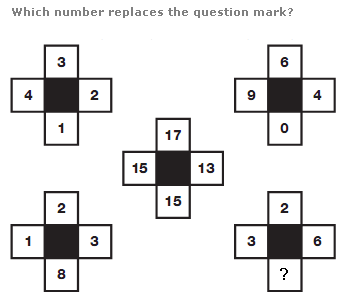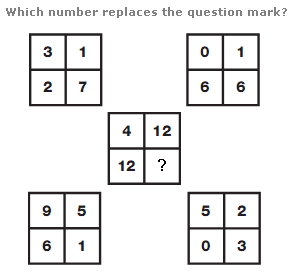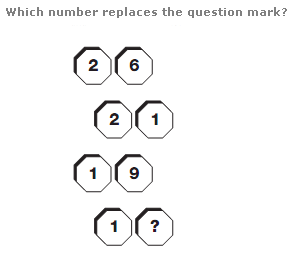# Puzzles - Number puzzles

### Exercise :: Number puzzlesAnswer : 6 Explanation : Take values in corresponding positions of the outer 4 shapes, and put the results in the central shape, rotating the position of this result by 1/4 turn each time.Answer : 9 Explanation : Calculate the sum of the numbers in corresponding positions of the outer 4 squares, subtract 5 form this result, and put it in the corresponding position of the middle square, 1/4 turn anticlockwise from the original.Answer : 6 Explanation : Working from top to bottom, reading each pair of numbers as a 2 digit value, values represent the reverse alphabetical value of letters of the alphabet, starting with A, written with 3 straight lines only.